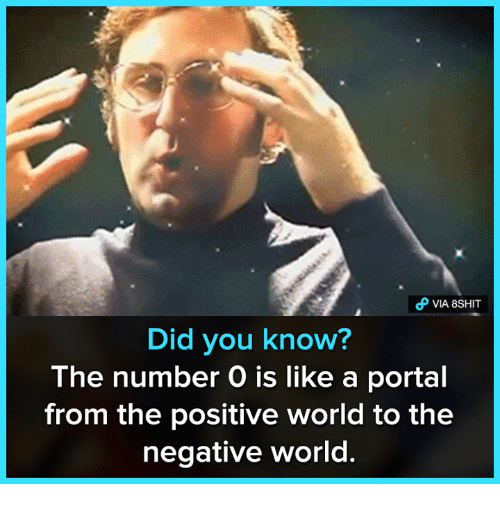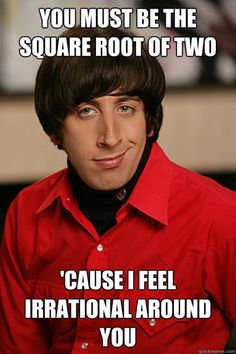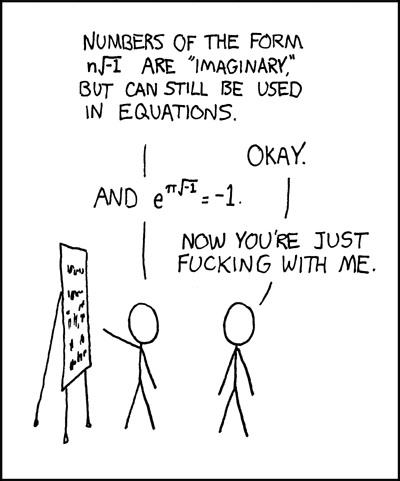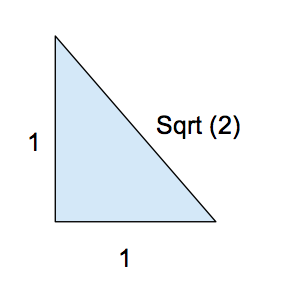Prompt Images

Pop quiz. Which of these numbers doesn’t belong?If you are like most people, you are probably thinking that last one is the most out of place. The square root of negative 1, also known as i to the cognoscenti. Because if a negative number multiplied by itself equals a positive number, then it’s hard to understand the square root of a negative number. This number, along with all possible multiples of it (i.e., 2 i , 3 i , 103.7457 i , etc.) make up the set of numbers that mathematicians call “imaginary numbers.”

Many people are uncomfortable with imaginary numbers, having a strong preference for the so called “real” numbers. This is not, as you might have hoped, due to a preference for keeping it real. It’s because when we are first introduced to imaginary numbers in an algebra class (more on that below), we can’t help but feel like they are a bit of a mathematical gimmick. Another unnatural invention of the coastal elites forced onto folks with more quaint sensibilities.In fact, imaginary numbers have been making people uncomfortable ever since the 16th century, when they were first formally introduced.1 Mathematicians of this period used all kinds of pejorative terms to describe them, such as false, nonsense, incomprehensible, and impossible, presumably declaring their objections in the fashion of Vizzini.So, are imaginary numbers legitimate numbers? Or just some kind of arithmetical tomfoolery?

You want the truth?

YES 👏👏 THEY 👏👏ARE 👏👏 LEGITIMATE 👏👏 NUMBERS 👏👏

To understand why they are legitimate, we have to go back and think about them in the context of all the others sorts of numbers that are more familiar to us.

How We Learn Numbers

The usual way of learning mathematics goes something like this (your mileage may vary):

1) First we learn about the “natural” numbers, like 1, 2, 3. They are by far the most intuitive numbers to understand. These are the numbers that our Fitbits use to count our steps.2) Then we are taught that there are more numbers: like 0, and also negative numbers: -1, -2, -3. These, together with the natural numbers, form the full set of integers.3) Then we learn that you can have “partial” numbers, or fractions, like ½, or 897/987. These are the rational That is, the set of all integers plus all the various possible ratios of integers.4) Then we learn that some numbers can’t be represented by fractions—these are irrational numbers, like √2. If you want to write this number out as a decimal, you better be prepared to keep writing digits forever. The set of all the rationals AND irrationals is called the real numbers.5) Only after learning about all those different kinds of numbers do we finally we learn algebra—that is, how to write equations for unknowns like x, and how to manipulate said equations to “solve for x.”6) And then one day our math teacher tries to pull a fast one by telling us we MUST open up our hearts and minds to i = √-1 because it’s the ONLY “number” that will solve the algebraic equation x2 = -1, EVEN THOUGH OUR PREVIOUS MATH TEACHER TOLD US TO NEVER, EVER, UNDER ANY CIRCUMSTANCES, TAKE THE SQUARE ROOT OF A NEGATIVE NUMBER BECAUSE WE MIGHT BREAK THE UNIVERSE.There’s a pedagogical reason for learning things in this order. I suppose it’s easier for kids to understand fractions than to understand the rules of algebra. But there’s another way to learn these things that leverages algebra way earlier in the process. Specifically, we can use algebra to define all those additional kinds of numbers in the first place.

A Different Way to Think About Numbers

Imagine we only know about the integers, but not any of the other number systems beyond the integers (like the rationals or irrationals). In addition, we also know about the rules of algebra, like how to write equations for unknowns (x) and manipulate them to get a solution.

Obviously, with the integers in hand, you can solve simple algebra problems like:

x + 1 = 0 (Answer: x = -1)

But what about a problem like 2x = 1? What’s the integer that solves this problem?

There isn’t one. To get a solution, you need to invent a new kind of number. Namely, the rationals (fractions). If you simply expand the world of numbers to include all possible ratios of integers (i.e., all the fractions), then ½ is now a legitimate number and your problem 2x = 1 has a legitimate numerical answer.2

Now the rationals are all well and good for solving algebraic equations with only a single power of x. But what about when you have equations with x2—whose solutions involve taking square roots? Some equations with x2 will still be solvable by integers or rationals.

x2 = 4 has a solution in the integers, namely x = 2.

But what about x2 = 2 ? There’s no rational number that would work as a solution here. To get a solution you need to invent a new kind of number. But what kind of number?

Easy, just invent a new number called √2 and define it to be the number that solves the above equation. BAM.What is that number, like written out in decimal form? Who cares. Let some other nerd worry about that!

The above trick works for inventing new numbers like the rationals (e.g., ½) and irrationals (e.g., √2 ). Will it also work for inventing imaginary numbers?

Yes.

Consider x2 = -1. There are no rational or irrational numbers that can solve this equation (you need real numbers with opposite signs to get a (-) sign, which is impossible if you are multiplying a real number with itself).

So to solve this problem you need to expand your number system yet again. And this is where we finally arrive at imaginary numbers. Define i to be a new “number” that, when squared, equals -1. 3

Honestly, we could do this all day. Creating new numbers for anyone who wants one.When you consider the numbers from this “algebraic perspective,” you see that the definition of imaginary numbers is completely consistent with the definition of the other kinds of numbers that we are more than happy to accept. In each example of numbers above, we used our existing number system and the rules of algebra to motivate inventing yet another kind of number whenever we ran into algebraic equations that couldn’t be solved with existing numbers.

But You Can’t Count to i

As we just saw, the logic of imaginary numbers is completely above board. The mathematicians have dotted their i’s and crossed their t’s, so to speak.

Of course there’s a difference between providing a formal definition of something and actually understanding it on an intuitive level.

Admittedly, with the exception of the imaginary numbers, each of the above sets of numbers has a straightforward intuitive interpretation. Obviously the natural numbers are associated with a certain “number” of objects, like goats or apples. Zero just means “no goats” or “no apples.” We can even think of ½ of an apple, so the rationals (fractions) have an intuitive interpretation as well.

Negative numbers are a bit trickier to explain this way, but most people are satisfied to think of them as representing a deficit of something—like (-3) goats means you owe me 3 goats.

What about the irrationals that can’t be represented as half an apple or 4/7 of a goat? Well, the Real Numbers, including both the rationals and irrationals, form a continuous set of numbers. These match up nicely with our intuition of things we can measure (length, time, etc.) to any arbitrary degree of precision.

But imaginary numbers do not have a comparable intuitive interpretation. There’s no obvious word problem to relate imaginary numbers to your 10 year-old’s life.What would it mean to have 3 i pieces of bacon, exactly?

You also can’t measure some continuous physical quantity with them, like you can do with the Real Numbers. I mean, imagine the absurdity of telling someone it’s 5 imaginary miles to the nearest gas station, or telling your friend you will be arriving in 10 imaginary minutes.4

So then maybe imaginary numbers really are odd ducks after all?

It’s not i, it’s you.

As I said before, I think the problem is less with imaginary numbers and more our intuition about numbers as a general category. As we are about to see, our intuitive concept of “number” is fuzzier than we realize.

Let’s forget about imaginary numbers for a moment and focus on the irrational numbers. Let’s take our example of √2. Now most people have no problem conceding that√2 is a number. It’s sort of a weird number, with its infinitely running decimal expansion, but it’s a number nonetheless. We can make intuitive sense of it. How? As the length of the hypotenuse of a right triangle with two other sides of length 1 (say 1 meter, but it works for any units).The equation for this triangle: 12 + 12 = (√2)2

You remember, right? The Pythagorean Theorem? From high school geometry class?In any case, that’s how the Greeks thought of these numbers—every (positive) number could be thought of as the length of some side of some triangle, and those sides were related by some formulas. (On the other hand, the Greeks didn’t like negative numbers. They reasoned it was impossible to have less than nothing of something, which is not an unreasonable objection.)

But wait. Has anyone ever actually measured a real triangle, in the real world, that has a length EXACTLY equal to √2? It isn’t actually possible, because you’d need a ruler with perfect precision to measure a length of 1.41421356237… where the numbers just keep going. Good luck finding that ruler at Staples!

Even when you picture in your mind this hypothetical triangle with a hypotenuse of √2, you aren’t actually picturing something with that precise length. You are picturing a hypotenuse of some undefined length and telling yourself it has length √2. And how do you know it has length if you can’t actually measure it? Because Algebra. That’s how.

In other words, you don’t have any real intuition for the number √2. At best you have intuition for a rational number that is sort of close to √2 — like 1.414.

To summarize, then, on one hand we have √2, a mathematical object that is defined by an algebraic equation, and for which we really don’t have a good intuitive understanding when we stop to actually think about it.

And on the other hand we have i , which we know is also defined by an algebraic equation, and for which we also really don’t have a good intuitive understanding.

And you are OK with being called a “legitimate” number, but not i ?Beyond Real-ism

At this point people who think imaginary numbers aren’t legitimate numbers have two options. Come up with a super narrow definition for “number” that rules out a bunch of other numbers that most people are OK with, to hold on to their claim that imaginary numbers aren’t legitimate. Or admit their notion of number is less consistent than they realized, and certainly less consistent than the notions put forward by people who do nothing but sit around and think about numbers all day.

If the latter, welcome to the world of woke math.

Where we acknowledge systematic realism in the world around us and where we are OK with numbers that don’t conform to our preconceived notions of what a number should be. For everyone else, your numerical fragility is showing, and it’s not a flattering look.

Footnotes:

1. Imaginary numbers even tripped up the great philosopher Rene Descartes (of “I think, therefore I am” fame)—who actually coined the term for these new mathematical objects. Here’s the description of imaginary numbers that Descartes gave in his work La Geometrie:

“For the rest, neither the false nor the true roots are always real, sometimes they are only imaginary, that is to say one may imagine as many as I said in each equation, but sometimes there exists no quantity corresponding to those one imagines.”

The best we can say of this pretzel twist of words is that it hopefully reads better in the original French.

1. Technically, you’ll need to first “define” the ratio ½ to be the “number” that, when multiplied by 2, gives 1.
2. Now a word of caution. We tend to *think* of i as being equal to √-1. But in fact it’s really defined by the equation i 2 = -1. This isn’t quite the same thing, since with real numbers we have the identity √a*√b =√(a*b), whereas if we were allowed to do this with imaginary numbers we’d get√-1*√-1=√(-1*-1) = √(+1) , which could actually be either -1 OR +1. So i isn’t actually identical to √-1, it really is a whole new kind of number that can’t be put together a la Frankenstein with existing pieces from the Real numbers. Also, this is why mathematicians prefer using the symbol i to √-1, to avoid making the above algebraic mistake.
3. Technically, there is something called “imaginary time” in physics. It was something Stephen Hawking liked to talk about to really blow people’s minds. But we’ll just put that one aside for now…
Jesse Stone

Jesse B. Stone loves science and writing. Apologies if you were looking for the "Jesse Stone" played by Tom Selleck in the CBS movies.